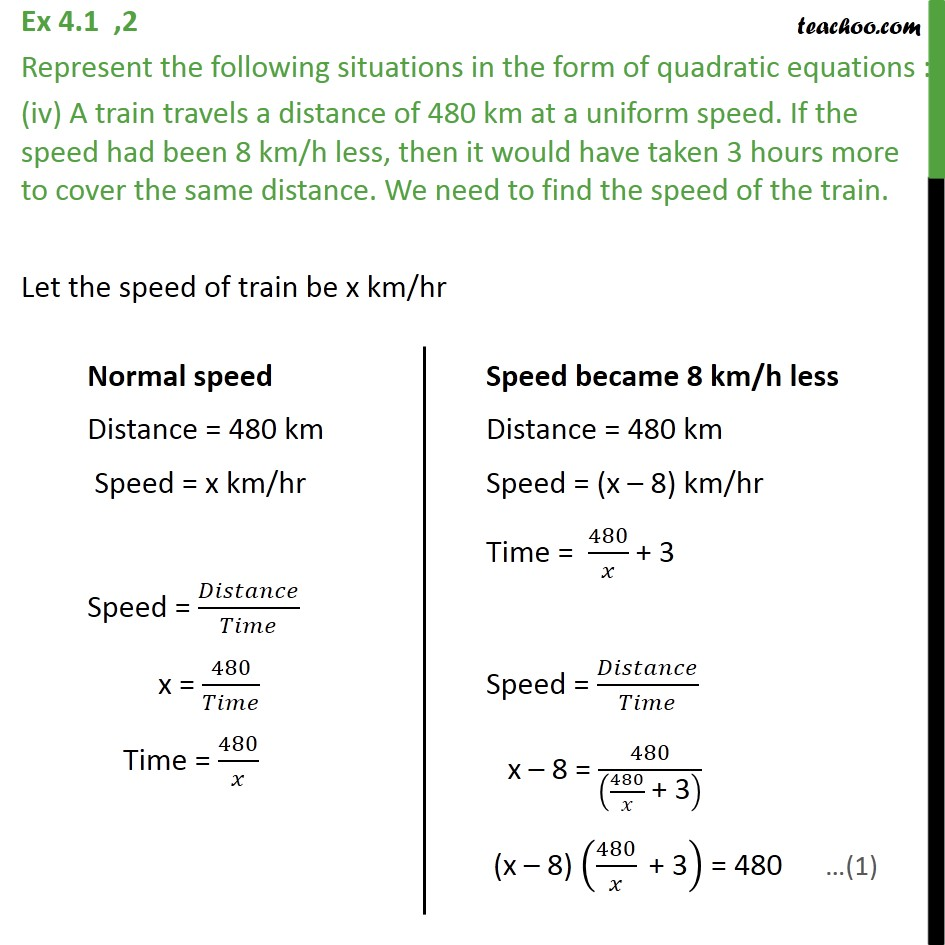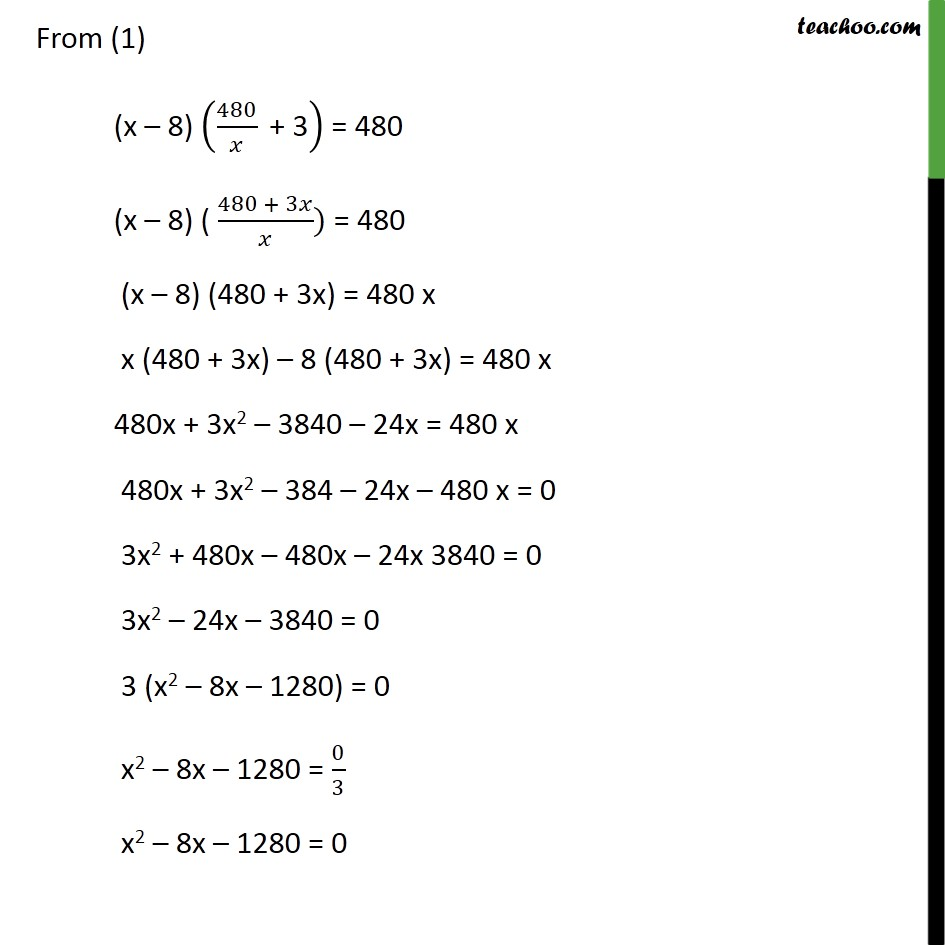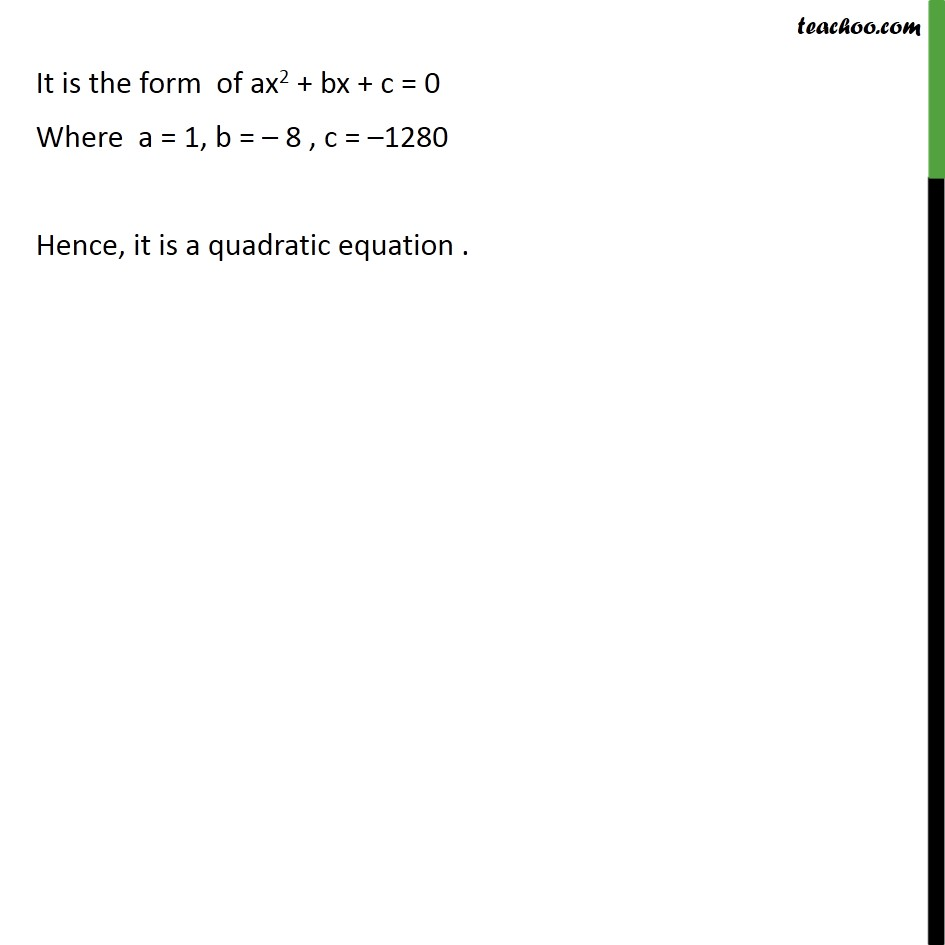Ex 4.1

Chapter 4 Class 10 Quadratic Equations
Serial order wiseLearn in your speed, with individual attention - Teachoo Maths 1-on-1 Class

### Transcript

Ex 4.1 ,2 Represent the following situations in the form of quadratic equations : (iv) A train travels a distance of 480 km at a uniform speed. If the speed had been 8 km/h less, then it would have taken 3 hours more to cover the same distance. We need to find the speed of the train. Let the speed of train be x km/hr From (1) (x 8) (480/ " + 3" ) = 480 (x 8) ( (480 + 3 )/ ) = 480 (x 8) (480 + 3x) = 480 x x (480 + 3x) 8 (480 + 3x) = 480 x 480x + 3x2 3840 24x = 480 x 480x + 3x2 384 24x 480 x = 0 3x2 + 480x 480x 24x 3840 = 0 3x2 24x 3840 = 0 3 (x2 8x 1280) = 0 x2 8x 1280 = 0/3 x2 8x 1280 = 0 It is the form of ax2 + bx + c = 0 Where a = 1, b = 8 , c = 1280 Hence, it is a quadratic equation .